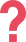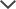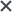## What is the Accuplacer College-Level Math Placement Test?

The ACCUPLACER College-Level Math placement Test evaluates your ability to solve problems that involve college-level mathematical concepts. The exam makes up one of the three math tests on the ACCUPLACER.

## ACCUPLACER College Math Placement Tests

Begin studying for the ACCUPLACER college math placement test with the ACCUPLACER Math Pack. Get hundreds of questions and detailed answer explanations for all three ACCUPLACER math tests. Upgrade to the ACCUPLACER Premium Pack for college placement testing practice.

## ACCUPLACER College-Level Math Test Topics

The College-Level Math section of the ACCUPLACER math test consists of 20 questions. These questions evaluate your abilities in five areas:

### 1. Algebraic operations:

• polynomials
• powers
• roots
• absolute value
• simplification and operations with algebraic fractions and expressions

### 2. Equations and inequalities:

• linear
• exponential
• other algebraic equations and inequalities

### 3. Coordinate geometry:

• the coordinate plane
• straight lines
• conics
• locus of points
• graphs of algebraic functions

### 4. Applications:

• translating verbal problems
• complex numbers
• series and sequences
• determinants
• permutations and combinations
• fractions and polygons

### 5. Functions & Trigonometry:

• exponents and logarithms including computations with functions and graphical properties
• periodicity, amplitude, and other properties
• trigonometric functions, identities, equations, and inequalities
• laws of sine and cosine
• graphs of trigonometric functions

## ACCUPLACER College-Level Math Sample Questions

Use our free questions below to begin your ACCUPLACER college math placement test practice.

### Question 1: Level 1 ACCUPLACER College-Level Math Question

The inequalityis equivalent to which of the following?

1.2.3.4.5.Scroll down to reveal the answer and explanation.

To solve this inequality, open the parentheses and simplify:The inequalityis equivalent to; therefore, the correct answer is (B).

If you chose answer (C), you probably reversed the inequality sign instead of following the rules—only reverse the inequality sign when you multiply or divide by a negative number.

If you chose answers (D) or (E), you probably divided byinstead of.

### Question 2: Level 2 ACCUPLACER College-Level Math Question

What is the value ofin the equation?

1.2. 3
3. 7
4. 10
5. 100

Scroll down to reveal the answer and explanation.

In order to solve this problem, apply these logarithm rules:Method A:Note that, thus:Useand find:Method B:Note that, thus:From the calculations; therefore, the correct answer is (E).

If you chose any other answer, review your calculations and logarithm rules.

### Question 3: Level 3 ACCUPLACER College-Level Math Question

Every Monday morning Brandon receives \$400 for the chores he did the week before. Each week he spends between \$300 and \$460. If Brandon has \$200 on Sunday evening, how much will he have saved three weeks later?

1. .\$0 - \$420
2. \$0 - \$500
3. \$20 - \$420
4. \$20 - \$500
5. \$420 - \$500

Scroll down to reveal the answer.

The answer is a range; therefore, find the maximum and minimum amount of money Brandon may have saved three weeks later.
In order for Brandon to have saved the maximum amount, he needs to spend the minimum amount—\$300.

On Sunday evening he had \$200.

Every week Brandon receives \$400 and spends \$300:

Earn(\$)=400−300=100

Three weeks later:, the maximum amount is \$500.

In order for Brandon to have saved the minimum amount, he needs to spend the maximum amount—\$460.

On Sunday evening he had \$200.

Every week Brandon receives \$400 and spends \$460:

Earn(\$)=400−460=−60

Three weeks later:, the minimum amount is \$20.

The maximum amount Brandon may have three weeks later is \$500, and the minimum amount he may have is \$20. Therefore, the correct answer is (D).

## ACCUPLACER College Math Placement Test Study Guide

Start your preparation with free college math questions for the ACCUPLACER exam. You can access and download our free booklet of questions and detailed answer explanations. The booklet includes 30 college English and math placement questions. To take a practice college math placement test now, simply download the ACCUPLACER PDF.Get Your Free ACCUPLACER Study Guide PDF Here

## Start ACCUPLACER College Math Placement Test Prep Now!

Don’t delay preparing for your ACCUPLACER math test. If you have to take a college math placement exam or ACCUPLACER math admissions exam, begin your ACCUPLACER prep now. Get questions and explanations to help you prepare for the ACCUPLACER College-Level math test.

ACCUPLACER® and WritePlacer® are trademarks and property of COLLEGE BOARD. The trademark holder is not affiliated with TestPrep-Online or this website.

Need HelpNeed Help